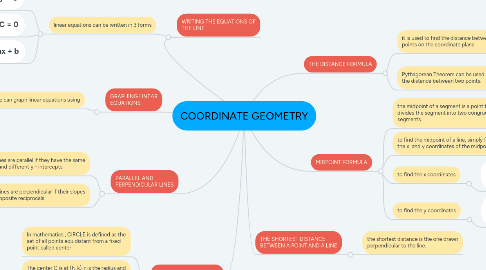# COORDINATE GEOMETRY

Get Started. It's FreeCOORDINATE GEOMETRY## 1. WRITING THE EQUATIONS OF THE LINE

### 1.1. linear equations can be written in 3 forms

1.1.1. Standard Form : Ax + By = C

1.1.2. General Form : Ax + By + C = 0

1.1.3. Slope - Intercept Form : y = mx + b

## 2. GRAPHING LINEAR EQUATIONS

### 2.1. we can graph linear equations using

2.1.1. x - and y intercepts

2.1.2. a point and slope of the line

2.1.3. two points of the line

## 3. PARALLEL AND PERPENDICULAR LINES

### 3.1. Two lines are parallel if they have the same slope and different y - intercepts

3.1.1. slope of L1 = slope L2

### 3.2. Two lines are perpendicular if their slopes are opposite reciprocals

3.2.1. the product of their slopes is always equal to -1

## 4. EQUATION OF THE CIRLE

### 4.3. the distance formula is used to find the equation of the circle

4.3.1. thus the equation of the circle whose center is at (h, k) and with radius r is

## 5. THE DISTANCE FORMULA

### 5.2. Pythagorean Theorem can be used to find the distance between two points.

5.2.1. The Pythagorean theorem is attributed to Greek mathematician PYTHAGORAS OF SAMOS

## 6. MIDPOINT FORMULA

### 6.3. to find the x coordinates

6.3.1. add the first and second value of x and divide it by two

### 6.4. to find the y coordinates

6.4.1. add the first and second value of y and divide it by two